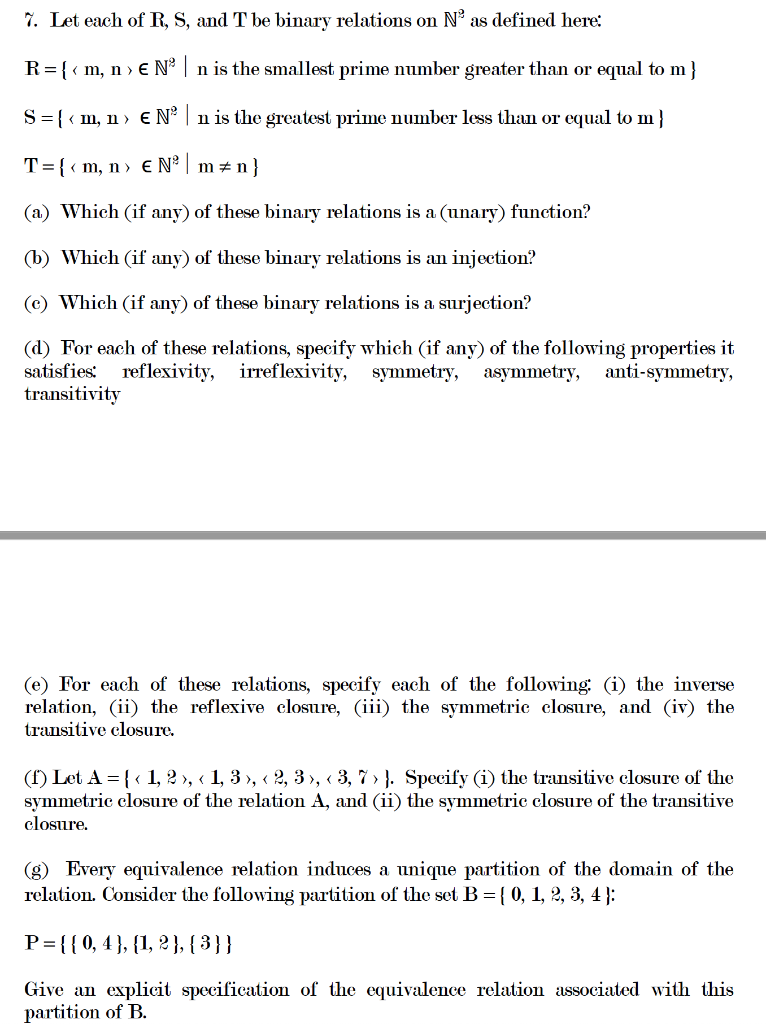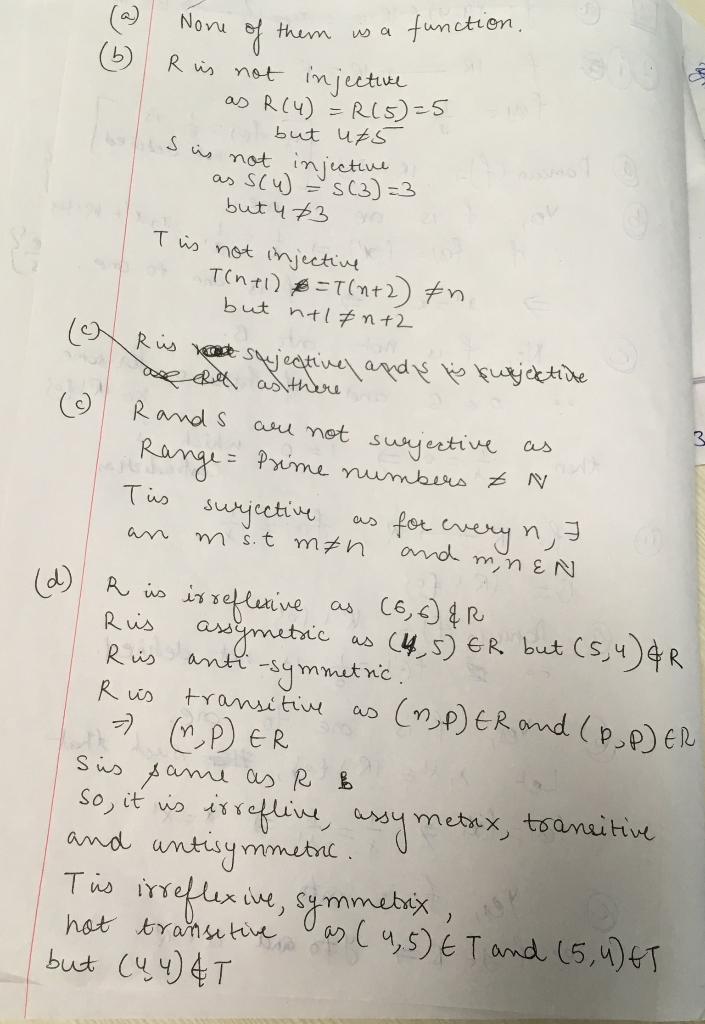Homework Help Question & Answers

# I. Let each of R, S, and T be binary relations on N2 as defined here: R-[<m, n EN nis the smallest prime number greater than or equal to m] S -[< m, n> EN* nis the greatest prime number less...I. Let each of R, S, and T be binary relations on N2 as defined here: R-[we are only supposed to do four parts in a question,please post y

##### Add Answer of: I. Let each of R, S, and T be binary relations on N2 as defined here: R-[<m, n EN nis the smallest prime number greater than or equal to m] S -[< m, n> EN* nis the greatest prime number less...
More Homework Help Questions Additional questions in this topic.

• #### 9x+5greater than or equal to -40 or 7x+3greater than or equal to -11 solve and graph I got the answer of x greater than or equal -5 or x is greater than or equal to -2 as the solution to this equations, but how do i put this into a soltuion set

Need Online Homework Help?

Get FREE EXPERT Answers
WITHIN MINUTES
Related Questions